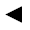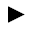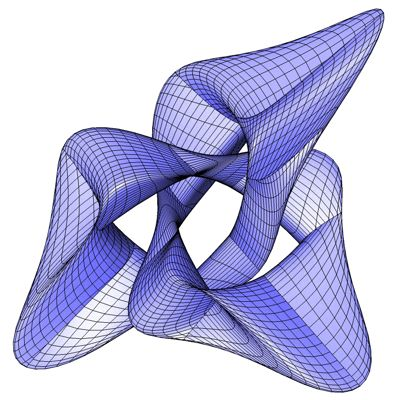п»їп»їп»ї math handbook calculator - Fractional Calculus Computer Algebra System software+++=п»ї[back]

## 3.53. Tranguloid Trefoil

[next]The tranguloid trefoil [ 8 ] is represented by the following equations.

 x = 2 sin(3u)/(2 + cos(v)) 3-178 y = 2 (sin(u) + 2 sin(2 u))/(2 + cos(v + 2pi/3)) 3-179 z = (cos(u) - 2 cos(2 u)) (2 + cos(v)) (2 + cos(v + 2pi/3))/4 3-180

To represent the area, the two parameters u and v can assume the following values ​​(domain of definition), for example.

 u is an element from the number set [-pi, pi] v is an element from the number set [-pi, pi]

Since the Tranguloid Trefoil is a closed figure, the definition range must be adhered to exactly, it cannot be changed with the plugin.Fig. 57